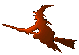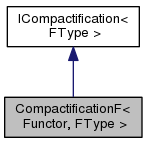Hex  1.0 Hydrogen-electron collision solver
CompactificationF< Functor, FType > Class Template Reference

Compactification of a function from finite interval. More...

#include <compact.h>

Inheritance diagram for CompactificationF< Functor, FType >:[legend]
Collaboration diagram for CompactificationF< Functor, FType >:[legend]

## Public Member Functions

CompactificationF (Functor f, double a, double b)
Constructor of the class. The parameters "a" and "b" specify original definition interval [a,b]. It must be a < b. More...

double scale (double x) const
Scale value from the original interval [a,b] into compactified interval [-1,1]. More...

double unscale (double t) const
Unscale value from the compactified interval [-1,1] into the original interval [a,b]. More...

double Jacobian (double t) const
Evaluate Jacobian of the transformation. More...

FType operator() (double t) const
Evaluate the compactified function. More...Public Member Functions inherited from ICompactification< FType >
virtual ~ICompactification ()

## Detailed Description

### template<class Functor, typename FType> class CompactificationF< Functor, FType >

Transform arbitrary function so that its definition range will be $$t \in [-1,1]$$, for original interval $$x \in [a,b]$$. The following formula is used:

$x = \frac{a+b}{2} + t \frac{b-a}{2} \Leftrightarrow t = \frac{x - (a+b)/2}{(b-a)/2} \ .$

## Constructor & Destructor Documentation

template<class Functor, typename FType>
 CompactificationF< Functor, FType >::CompactificationF ( Functor f, double a, double b )
inline

## Member Function Documentation

template<class Functor, typename FType>
 double CompactificationF< Functor, FType >::Jacobian ( double t ) const
inlinevirtual
template<class Functor, typename FType>
 FType CompactificationF< Functor, FType >::operator() ( double t ) const
inlinevirtual
Parameters
 t Value from the compactified interval [-1,1].

Implements ICompactification< FType >.

template<class Functor, typename FType>
 double CompactificationF< Functor, FType >::scale ( double x ) const
inlinevirtual
template<class Functor, typename FType>
 double CompactificationF< Functor, FType >::unscale ( double t ) const
inlinevirtual

The documentation for this class was generated from the following file: